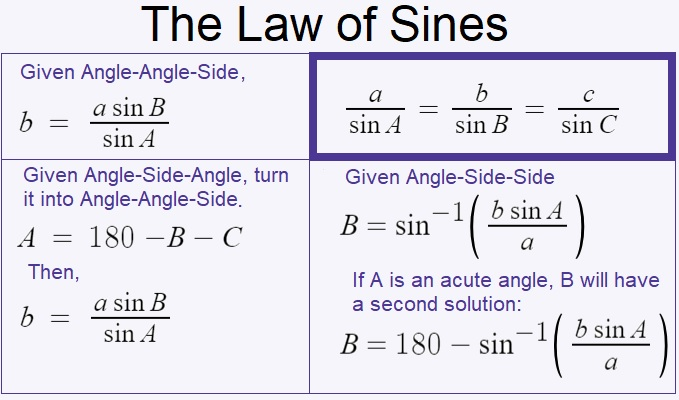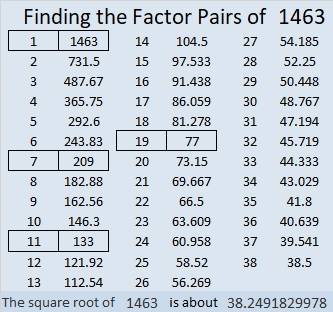# 1463 When to Use the Law of Sines and the Law of Cosines

For years I have taught my students the law of sines and the law of cosines using only a hand-held scientific calculator. Unfortunately, many of them don’t have access to one during the pandemic. I found some online law of sines and law of cosines calculators, but I felt like they all seemed to find solutions by magic, without requiring any understanding. I want my students to understand when to use the law of sines and when to use the law of cosines.

Contents

### When Should I use the Law of Sines?

Use the law of sines when you are given these three pieces of information about a triangle: Angle-Angle-Side, Angle-Side-Angle, or Angle-Side-Side. Here’s a graphic I made showing each case and how the law of sines is transformed to obtain the missing angle or side:I used Desmos to make a Law of Sines calculator that uses the above equations derived from the law of sines. Angles will be entered and given in degrees, not radians. This Law of Sines calculator still forces you to think about what information you’re given and which equation you should use. In your problem, there will be a known angle and a known side that are opposite to each other. Label these known values A and a, respectively. If you have another angle, label it B. If you have another side, label it b. Find the appropriate equation in the calculator, replace the variables with their values, and Desmos will calculate the answer. If you’re given Angle-Side-Side, you will need to use the inverse sine function, and you might have two solutions. Thus, if the given angle is acute, be sure to replace “Bacute” with the acute angle found in the formula above it so that you will also have that second solution. A triangle can’t have two obtuse angles, so don’t give two solutions for B if A is obtuse!

Some people might try to add sliders to that calculator because then they won’t have to think as much. Adding sliders would confuse Desmos with too many variables, so I also made a law of sines calculator with sliders. Angles will be entered and given in degrees, not radians. In your problem, there will be a known angle and a known side that are opposite to each other. Label these known values A and a, respectively. If you have another angle, label it B. If you have another side, label it b. Instead of typing the values into the equation, you can type the values in as sliders. The default for all the sliders is set at 0, so if any of your solutions is 0 or undefined, you are either looking in the wrong place for your answer or you haven’t entered enough information. If you use that calculator, you will need to refresh and reload it after each problem.

### When Should I use the Law of Cosines?

The Law of Cosines is easy enough to type: c² = a² + b² – 2ab cos C. It looks a little like the Pythagorean Theorem, and when C is 90º, that’s exactly what it is.

Use the law of cosines when you are given these three pieces of information about a triangle: Side-Angle-Side or Side-Side-Side. Here’s a graphic I made showing both cases:Since the Law of Cosines requires both a and b to be entered twice, I decided to go ahead and use sliders in the Law of Cosines calculator to make typing a little easier. Angles will be entered and given in degrees, not radians. If you are given Side-Angle-Side, let the angle be C. If you are given Side-Side-Side, label the side opposite the angle you are looking for, c. It doesn’t matter which side you label a or b, but that opposite side must be c. You will need to use the inverse cosine function, but I already have that in the calculator for you. You will need to refresh and reload before starting a new problem.

Since this is post number 1463, I’ll write a little bit about that number.

### Factors of 1463:

Since both 14 and 63 are multiples of 7, we can be sure that 1463 is divisible by 7.

• 1463 is a composite number.
• Prime factorization: 1463 = 7 × 11 × 19
• 1463 has no exponents greater than 1 in its prime factorization, so √1463 cannot be simplified.
• The exponents in the prime factorization are 1, 1, and 1. Adding one to each exponent and multiplying we get (1 + 1)(1 + 1)(1 + 1) = 2 × 2 × 2 = 8. Therefore 1463 has exactly 8 factors.
• The factors of 1463 are outlined with their factor pair partners in the graphic below.Since all four of the factor pairs of 1463 contain only odd numbers, 1463 can be written as the difference of two squares based on those four ways:

732² – 731² = 1463 (732 is the average of 1 and 1463, and 731 is one less.)
108² – 101² = 1463 (108 is the average of 7 and 209, and 101 is seven less.)
72² – 61² = 1463 (72 is the average of 11 and 133, and 61 is eleven less.)
48² – 29² = 1463 (48 is the average of 19 and 77, and 29 is nineteen less.)

## 2 thoughts on “1463 When to Use the Law of Sines and the Law of Cosines”

1.Steve Morris

Good tips. My youngest son is working on this for his mathematics exams right now.

•ivasallay

These are certainly not the easiest times to master concepts in any subject. I would love to get your son’s feedback on these tips, and I wish him the best in ALL of his exams.

This site uses Akismet to reduce spam. Learn how your comment data is processed.